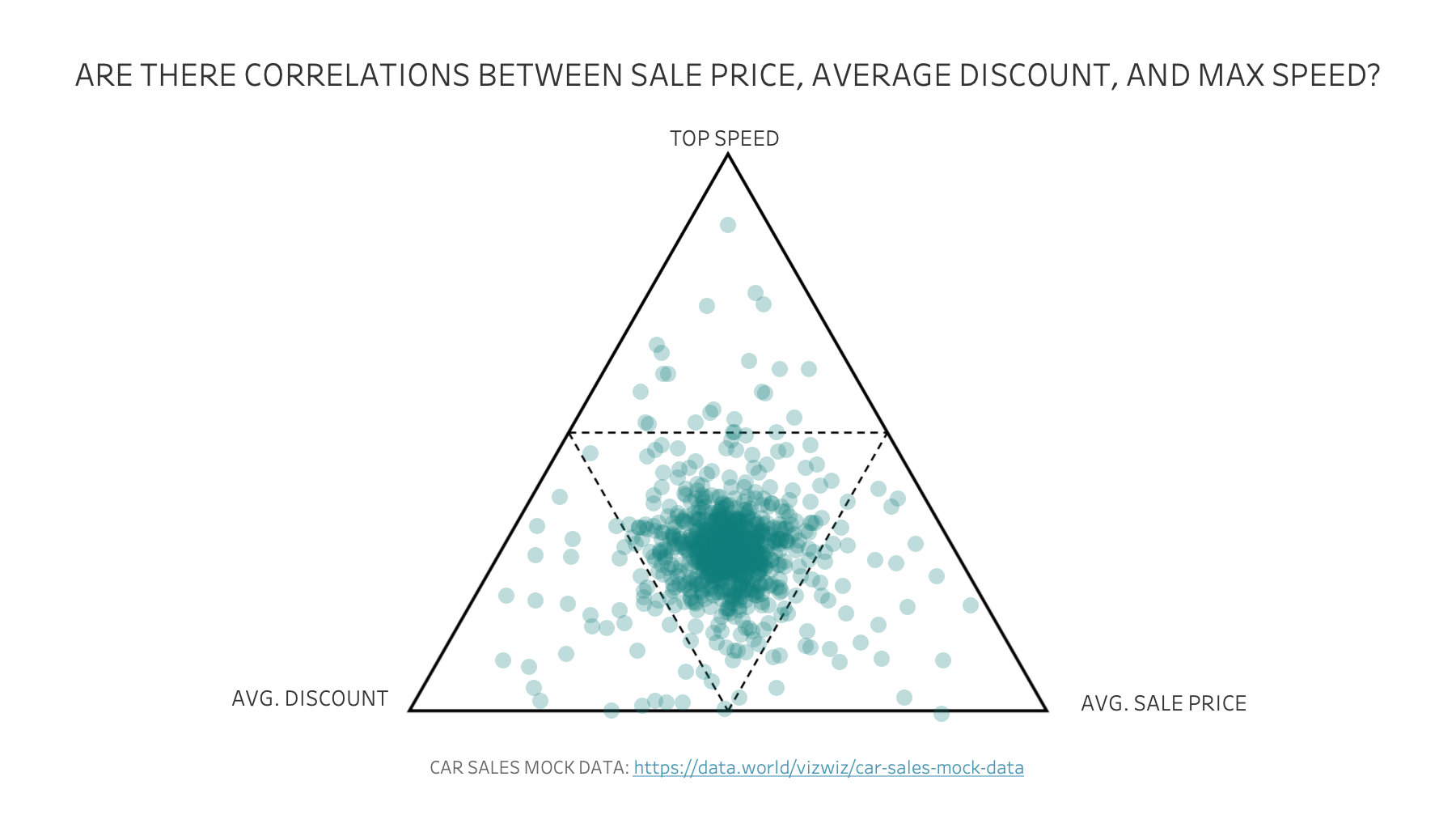## VizWiz

Data Viz Done Right

# How to Create a Ternary Graph / Triangular ChartTernary graphs visualise the ratios between the three variables. A ternary graph requires three metrics, plotted as a triangle, where the sum of all three variables adds up to a constant. You can think of it as a three dimensional scatterplot.

Each dimension is plotted based on its relative variance (on a scale of 0%-100%) to the largest value within the dimension.

A value plotted near the top would indicate a weighting towards the variable at the top. Likewise, for the bottom right, a value plotted there would indicate a weighting towards the variable that was plotted on the bottom right. A value in the middle, indicates the dimension is balanced across all three variables.

Download the Car Sales mock data set here. Download the triangle I used as the background image on the chart here.